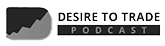# How to Use Forex Fibonacci Retracement LevelsForex traders have a difficult task: to know where the price goes next. For this, they use both technical fundamental analysis. Fibonacci retracement levels and the rest of the Forex Fibonacci tools form the basis of almost any trading theory.

Starting with basic support and resistance levels and ending with complex theories like the Elliott Waves Theory, the Fibonacci patterns are everywhere. Like it or not, technical analysis cannot exist without Fibonacci ratios/numbers.

Knowing something about what happened in the past helps traders forecasting the future. For this, the Forex Fibonacci ratios are great tools.

Confluence areas where multiple Fibonacci retracement levels form end up in strong support and resistance levels. The Golden Ratio (61.8% level) attracts both buyers and sellers in a bullish/bearish trend. Moreover, Fibonacci extensions in impulsive waves, and not only, tell traders where to exit/enter a trade.

What makes Fibonacci numbers so special? Why trading theories depend on these ratios?

Think of the Elliott Waves Theory, for example. Without the Fibonacci levels, Elliott couldn’t write down the rules of his cycles.

Multiple books were written on the importance of the Fibonacci sequence. Some showing complicated approaches, some more simplistic ones.

However, this article aims at showing different ways to use the Forex Fibonacci levels. Moreover, the idea is to look how trading with Fibonacci works, using simple and effective trading methods.

Keep in mind though that Fibonacci numbers are virtually everywhere around us. They surround our lives. But, there’s no place like Fibonacci in Forex technical analysis that shows how human behavior reacts around these levels.

# The Most Important Fibonacci Retracement Levels

The first thing that comes to mind is the golden ratio. In technical analysis, the 61.8% Fibonacci retracement gives the entry or exit point for many traders.

For example, in the Elliott Waves Theory, traders hunt impulsive waves. For this, they wait for a five-wave structure to unfold, and then for the price to retrace 61.8% of it.

When this happens, they take a trade. This is the most popular trade example with retail traders that use Elliott for their trading.

Because everyone knows that, we won’t mention it anymore in an impulsive structure. A Forex Fibonacci sequence is more important for technical traders.

Any trading platform offers the Fibonacci tools. They even have a dedicated button on the most popular trading platform (MT4).

Besides the Fibonacci retracement levels, the sequence appears in extensions, time zones, arcs, and so on. Again, there’s no area in technical analysis that deals with pattern recognition approach that doesn’t use a Fibonacci retracement calculation.

A Fibonacci retracement Forex tool is easy to use. Simply click and drag from the start to the end of a move and the trading software automatically projects the levels.

23.6%, 38.2%, 50% and 61.8% are the most important ones. However, depending on the trading theory used, Fibonacci retracement and extension levels vary greatly.

For instance, the 80% level plays an important role when trading with the Gartley 222 pattern. The 123.6% and the 138.2% Forex Fibonacci levels help traders identifying flat patterns with the Elliott Waves Theory. And, the list can go on.

## How to Use Fibonacci Retracement Levels

The classical way to use the retracement tool starts from two steps:

• Find a market advance or decline.
• Click and drag the Fibonacci retracements and extensions

A good question here would be what this has to do with the extension levels. Well, some patterns can retrace more than 100% of the previous swing.

For example, Elliott found that an irregular flat pattern MUST retrace between 100% and 123.6% of the previous swing. Therefore, only the classical Forex Fibonacci levels won’t help.

However, the classical way to use the Fibonacci retracement levels is to look for price to hesitate around them. If there was a bearish trend and the market bounces, traders use them to sell into resistance.

Take the chart below as the perfect example. When the European Central Bank (ECB) started to cut the interest rates, the Euro fell.

Yet, at some point in time, it found a bottom. And then, it jumped. Where?

You guessed it already: to the first Fibonacci retracement level. To this day, selling the 23.6% level proved to be a great Fibonacci retracement strategy.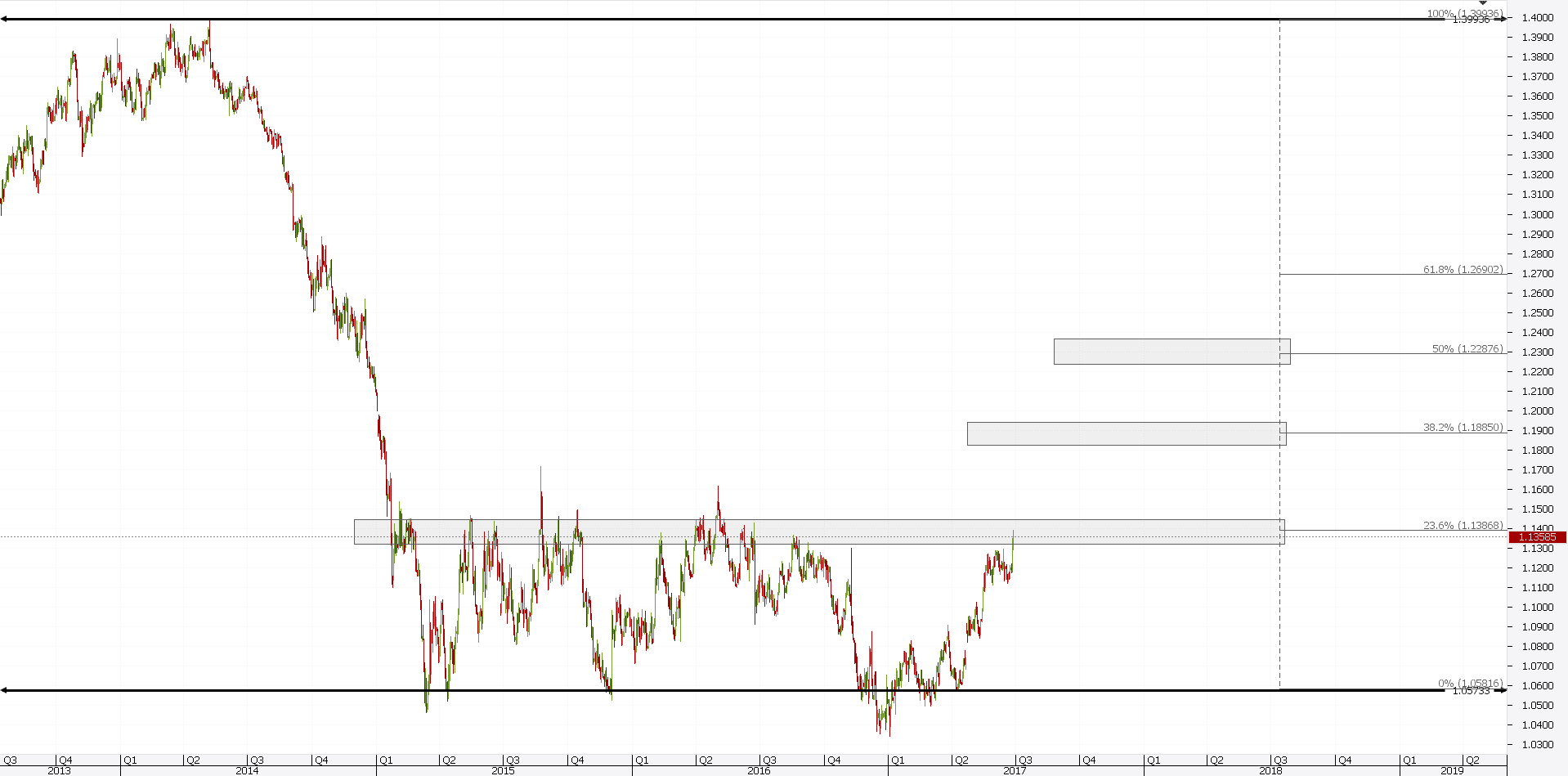However, bulls keep trying. The more the level gets tested, the less likely to hold further. As such, look for a Forex Fibonacci level to offer resistance/support two times.

If the price keeps trying, it will give up eventually. In this case, after two opportunities to sell the EURUSD pair at 23.6%, the third bounce from the absolute lows where great buying opportunities.

Moreover, this bullish Forex Fibonacci strategy should have the take profit at the 23.6% area. Next, traders simply should wait if the level holds. If not, the 38.2% is the next logical target.

## Forex Fibonacci Levels in Zigzags

A great Fibonacci Forex trading strategy uses the Fibonacci retracement levels in a zigzag. As a rule of thumb, the b-wave in a zigzag cannot end beyond the 61.8% level.

This gives traders a Forex Fibonacci strategy that works all the time. Let’s have a second look at that EURUSD chart.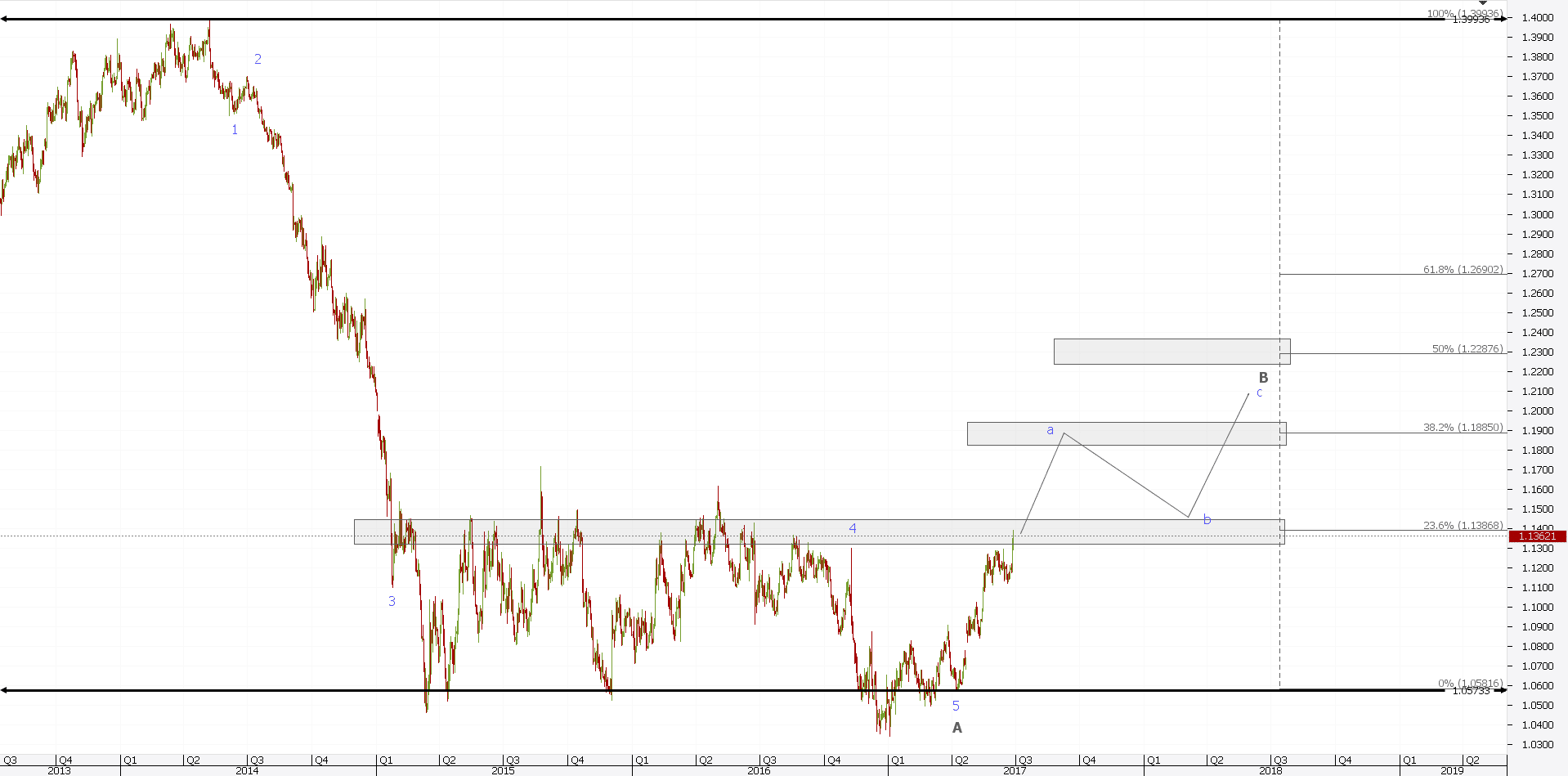A zigzag is a three-wave structure. The whole drop from 1.40 to almost parity is an impulsive wave, only the A-wave in black of the overall pattern.

Therefore, if we were to use the logic from a bit earlier, the 23.6% resistance is impossible to hold this time. The price has a scope to move further to 38.2% and beyond. But, in doing that, it meets strong resistance given by the Fibonacci retracement indicator.

The next step in this Fibonacci Forex strategy is to use pending orders. That is, pending sell limit orders.

The best Forex Fibonacci area is between the 50% and 61.8% levels. Hence, divide the area into four equal levels and place pending orders to sell for 38.2% and even 23.6%.

Fibonacci day trading techniques like this one work on any time frame. However, the key is from where to draw the Fibonacci retracement tool.

When the Fibonacci trading system doesn’t consider the highs and the tops of a move, levels differ. This is the case with the EURUSD example from above.

The 5th wave doesn’t end at the absolute low. Hence, the resulting Fibonacci retracements differ.

# How to Use Fibonacci Retracement in Forex Trading

When buying or selling a currency pair, traders look for the best opportunities they can find. After all, trading is a game of probabilities.

There’s no holy grail when it comes to Forex trading. As such, managing risk matters the most.

However, the idea is to enter a trade when the risk is minimum. Not to take a trade and keep the risk limited.

Forex Fibonacci levels help to define the minimum risk. That is especially true when traders use a pattern recognition approach.

Take harmonic trading, for example. Gartley defined the concept around the 80% Fibonacci retracement level.

However, it wasn’t enough. Pesavento came and introduced the 61.8%, 75% and even the 38.2% level in harmonic trading.

Yet, technical analysts wanted more. As such, Carney’s approach to harmonic trading stands the test of time. ALL Fibonacci retracement levels must be respected for the classical harmonic patterns as we know them today to make sense.

The same is with Elliott Waves Theory. Fibonacci Forex traders often find themselves in a clearly defined situation.

The price of a currency pair simply CANNOT move beyond a specific Fibonacci ratio. Hence, it gives the stop loss level.

Fibonacci retracements and extensions levels define technical analysis as we know it. However, the Fibonacci tool on any trading platform offers more than just retracement and extension levels.

Fibonacci arcs and the Fibonacci fan tools combine the ratios in different ways. However, they aim for the same thing: to find out support or resistance levels in a predetermined move.

If you’re familiar with the support and resistance levels provided, then you know how to use Fibonacci fan. In fact, the Fibonacci fan indicator is not that popular among Forex traders.

## How to Use Fibonacci Forex Pivotal Areas

Before learning how to use a pivotal area, we must learn how to define one. For this, traders use different Fibonacci retracement levels.

The idea is to measure two different moves (one bullish and one bearish, or the other way around). Next, look for the golden ratio levels on both moves to be significant.

Further, find two Forex Fibonacci levels that have a small/very small distance between them. In fact, look for a Fibonacci confluence area.

When all the factors above align, the market formed a pivotal area. That is one of the most powerful trading tools in technical analysis.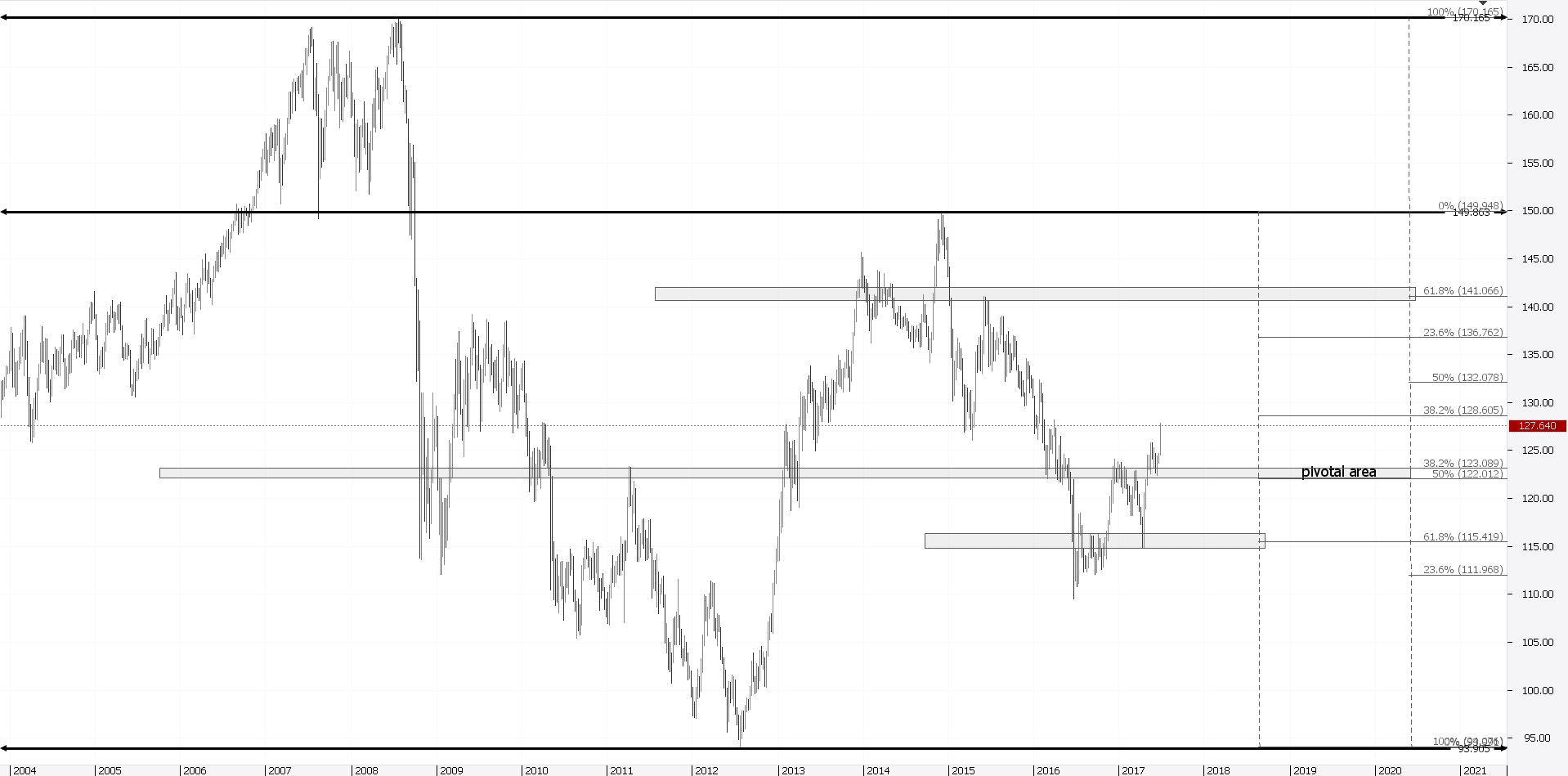Above is the EURJPY weekly chart. While the time frame is a big one, don’t get scared. When it comes to support and resistance levels, the bigger the time frame, the better.

Starting from left to right, the first move the market makes is bearish. We simply look for the 61.8% Forex Fibonacci level. The market hesitates and rejects from it.

Because of that, we start looking for a possible pivotal area. Next, we measure with another Fibonacci retracement tool the swing higher. Again, the market reacts from the resulting 61.8% level.

Finally, look for two Fibonacci levels close to one another. That’s the pivotal area.

If you look back in time, you’ll see the market respected it religiously. As such, look for the price to break above.

It did, after a perfect bounce from the 61.8% level. Such a Fibonacci retracement day trading strategy works every time. The key is to follow the rules.

Where do you think the price will move next? Providing the pivotal area holds, the next level of interest is the upper 6.8% Forex Fibonacci level. That one is more than a thousand pips higher from current levels.

And now I will show you a video example of how to take advantage of Fibonacci zones. The video below will show you the way a Fibonacci zone could appear to be a turning point on the chart. The video shows a price interaction with the 61.8% Fibonacci level, which leads to a bounce from the area and to a new impulse in the direction of the previous trend. This created a nice trading opportunity on the chart, which I took advantage of.

# Fibonacci Trading Strategies with Elliott Waves Theory

The Elliott Waves Theory cannot exist if it weren’t for the Fibonacci sequence. So important these levels are, that all the theory’s rules use them.

Here are some of the most important Fibonacci extensions and retracements levels in Elliott Theory rules:

• In an impulsive wave, at least one wave needs to extend. But, the extension means the wave is bigger than 161.8% of the previous wave.
• The b-wave in a flat pattern MUST end beyond 61.8% Fibonacci retracement when compared with the previous a-wave.
• At least three legs of a contracting triangle must retrace 50% of the previous leg.
• The b-wave in an irregular flat is smaller than 138.2% when compared with the previous a-wave.
• The un-extended waves in an impulsive move related to the golden ratio.

The list above is just to show the importance of the Fibonacci numbers in the theory. We can go on like this with pages of different rules. However, it will only highlight the strong dependency between the Fibonacci retracement levels and the Elliott Waves Theory.

But the Elliott Waves Theory doesn’t use the Forex Fibonacci levels only to refer to price, but also to time. The now famous rule of equality in an impulsive wave uses both price and time related to the golden ratio values.

Elliott found that every pattern uses Fibonacci levels. In fact, he defined the patterns based on different Fibonacci levels. That is, how to trade each pattern.

## Fibonacci Retracement in Triangles

Triangles are powerful patterns. Price spends most of the time in consolidation areas. As such, triangles form very often.

Elliott found that there’s a strong connection between the legs of a contracting triangle and different Fibonacci levels. All you should know is the length of the a-wave. Or, the first leg of the triangle.

One of the rules regarding triangles is that at least three legs must retrace more than fifty percent of the previous leg. If the triangle is bullish, all traders must do is to find that level and trade long every time the price reaches it.

Below there’s the AUDUSD daily chart. You can’t miss that triangle, no matter how hard you may try.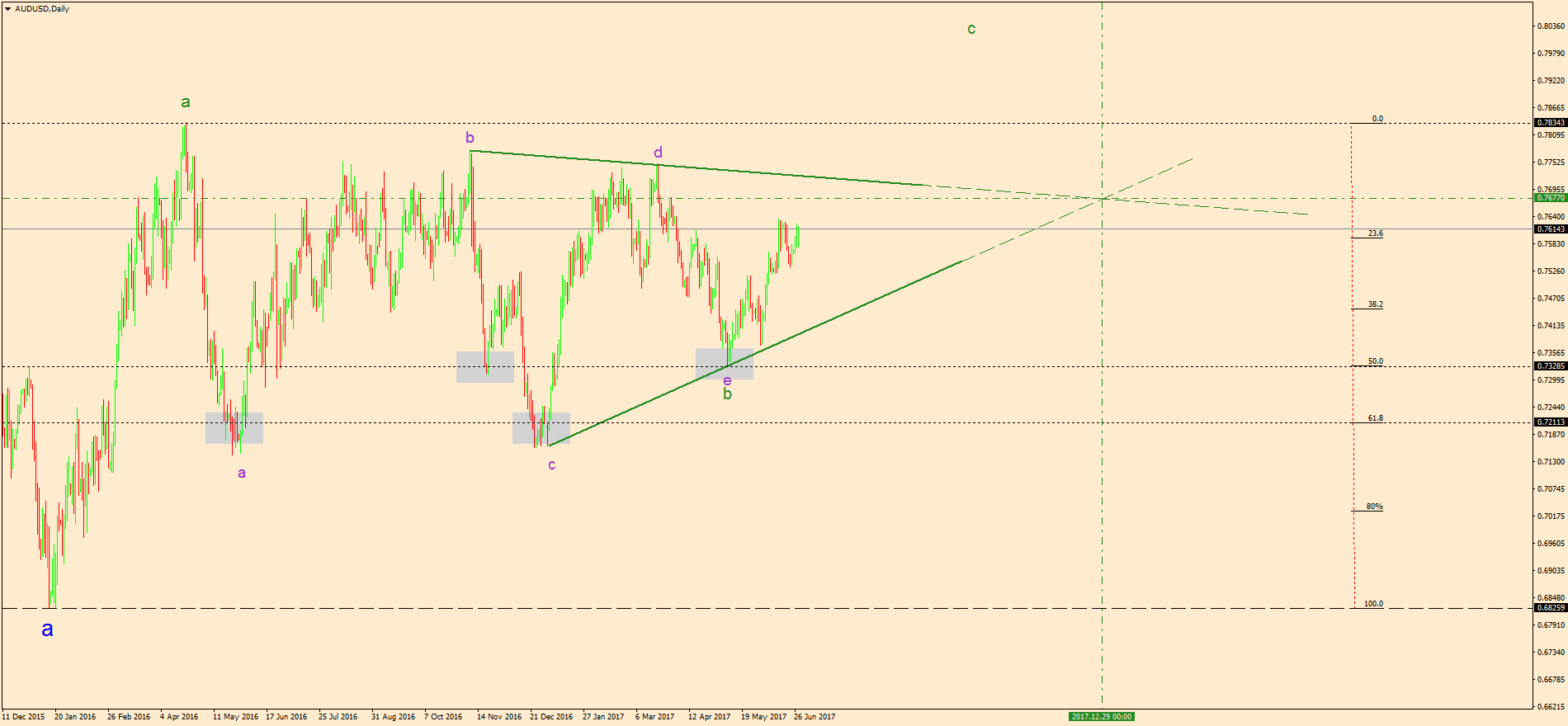A simple look at it tells you the bias is bullish. Because of the higher lows series, traders have a bullish bias.

A sound Forex Fibonacci approach is to measure the length of the a-wave with a Fibonacci retracement tool. The idea is to find the 50% and 61.8% levels.

In any triangle, the focus stays with the b-d trend line. The moment it gets broken, the triangle ends.

Until then, traders can scalp/swing trade their way in the triangle. As such, buying 61.8% and 50% retracement areas for the b-d trend line’s break will do the trick.

In this case, the trading strategy offered little or no retracement. That’s truly remarkable.

## How to Use Fibonacci Time Zones

One of the less known Forex Fibonacci indicators is the Fibonacci time zones one. It appears together with the other Fibonacci tools. The only difference is that it refers to time, not price.

Elliott Waves Theory is the only trading theory that allows traders to incorporate time to an analysis. Because of this, a sound Elliott Wave forecast values more than other trades.

The EURAUD chart below shows an example of how to use the Fibonacci time zones indicator. In an impulsive wave or a five-wave structure, the time zones indicator gives the estimated time the 4th wave will take.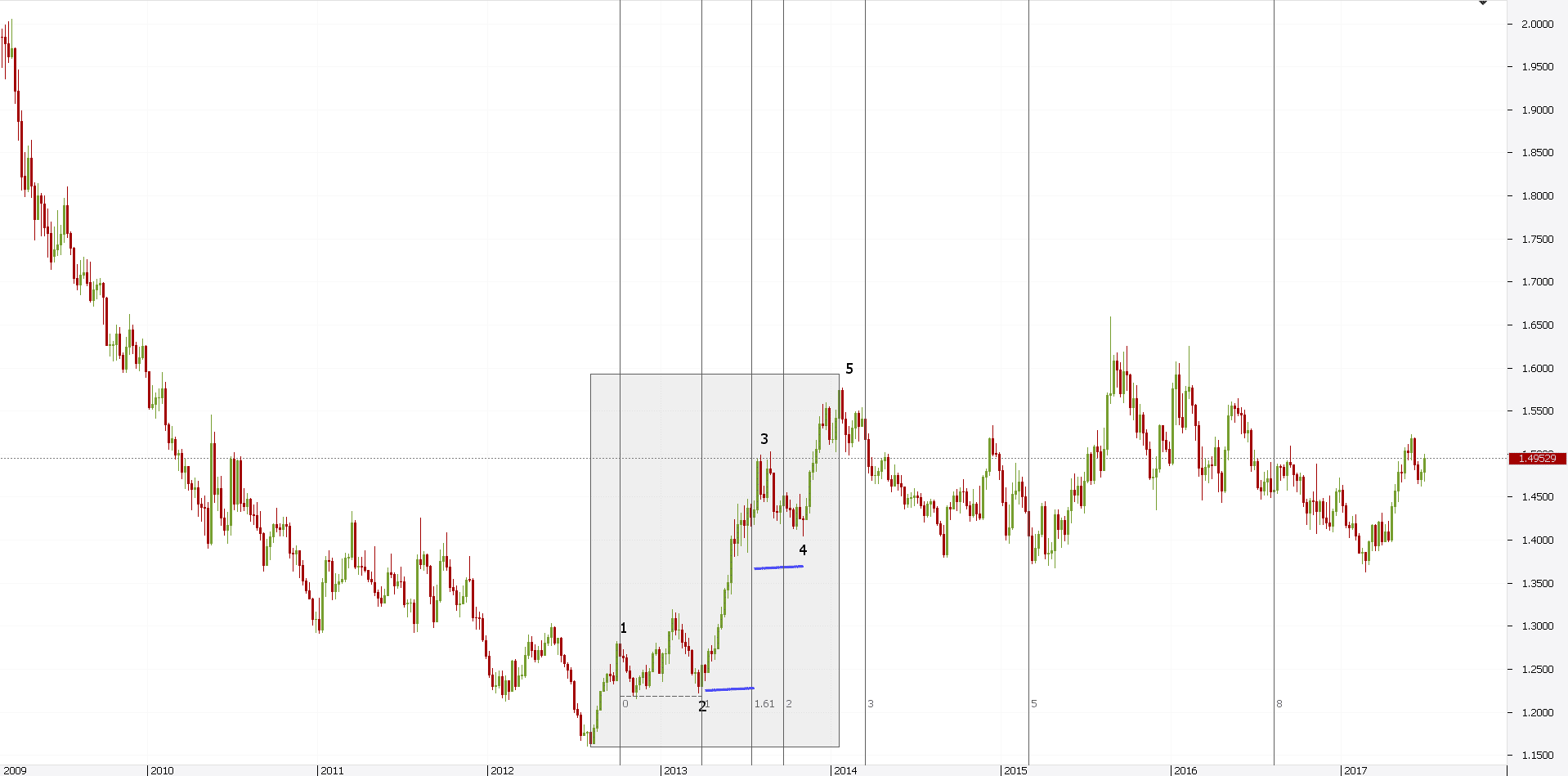In most cases, this will take 61.8% of the time taken for the 2nd wave. This way, not only traders know the price, but also the estimated time when the price will come.

The way to find this simple. On the MT4 trading platform, select the Fibonacci time zones tool from the dedicated Fibonacci tab. Then, simply click at the start of the 2nd wave. Next, drag the tool until the end of it.

If the 161.8% Fibonacci Forex level doesn’t appear, you can Edit the tool and add it. Finally, take the 61.8% measurement (the blue line in the chart above) and place it from the end of the 3rd wave.

The result will give an educated guess about the 4th wave time. There’s no rule related to the 4th wave price retracement.

However, using time, traders can “time” the moment they go long for the 5th wave.

# Conclusion

The Fibonacci retracement levels represent the most used Fibonacci tools in technical analysis. Different trading methods have different ways of how to draw Fibonacci retracement levels.

Some argue that the best way to use them is from top to bottom. Others argue that they work best from the start of a move until its end.

However, what if the move doesn’t end at the bottom? Moreover, what if it doesn’t start from the top?

This article’s purpose was to show you how to use different Fibonacci Forex levels to your advantage. However, the subject is much more complicated and extensive.

The examples here were intended to bring a new light to trading Fibonacci retracement levels. Moreover, they offer a new approach to the already known Fibonacci trading styles.

To sum up, Fibonacci numbers and levels are everywhere in technical analysis. Traders job is to make sure they use them correctly. For this, the tips and tricks shown here help to take the right trading decision.

What are you waiting for?

## START LEARNING FOREX TODAY!Damyan Diamandiev

Damyan is a fresh MSc International Management from the International University of Monaco. During his bachelor and master programs, Damyan has been working in the area of financial markets as a Market Analyst and Forex Writer. He is the author of thousands of educational and analytical articles for traders. When being in bachelor school, he represented his university in the National Forex Trading Competition for students in Bulgaria and got the first place among 500 other traders. He was awarded a cup and a certificate at an official ceremony in his university.

What are you waiting for?

## START LEARNING FOREX TODAY!

as seen on:
••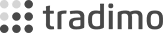••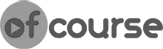•